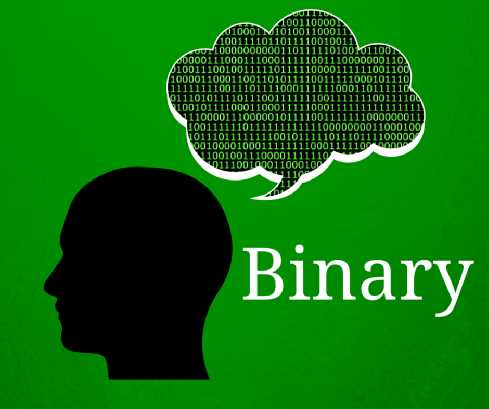# Binary Number System

There are four different number system used today in mathematics; binary number system, decimal number system, hexadecimal number system, and ASCII number system. This post is going to explore each of the aforementioned numbers of systems.
Binary number system
The binary number system is used in digital electronics and mathematics and is simply a number expressed in the base-2 binary numeral system, and uses only two symbols – zero (0) and one (1). So in simple terms, a binary number is a flow of information only in the form of "0" and "1" used by digital systems and computers.
The principles of binary systems are similar to all other numbering systems.
in the binary system, every number can be expressed by a sequence of bits or binary digits, which may then represented by any mechanism that is capable of existing in two mutually exclusive states.
The term bit is used commonly in binary numbering to mean the smallest unit of alphanumeric technology, and it stands for “BInary digiT. Eight bits make up a byte, while 1,024 bytes or 8,192 bits make up a kilobyte.
In this numbering format, 2 doesn’t exist and so, 1 + 1 = 10.
The binary system is preferred by many computationists due to its simplicity. It helps create any computing device with a series of switches that can alternate between an "on" and an "off" position. Provided these switches can be moved from one position to another on command, they can be mechanical, electrical, or biological. Try this and this binary translator for binary to other number system conversions.
When the switch is in an “on” position it represents the value of one, and when it is on an “off” position, it represents the value of zero.
Majority of electronic devices have switches, which they use to perform mathematical functions by turning it on and off. Therefore, the speed of operations by a computer is based on how faster it can turn the switches on and off.
Binary numbers can also be calculated using the same order of operations used to manipulate decimal numbers, but by using only 0 and 1. To add two binary numbers, you must follow four rules:
For example, using the example above, 1 + 1 = 10
This guide about binary number systems is very help full.
To come to the above conclusion, start in the right-hand column of the equation. Write the 0 and carry 1. Continue moving from right to left while you add until the problem is completely solved.

Last updated:11/22/2019 10:40:36 AM#### Arslan Fazal

Hello i am web designer and i have excellent experience, i am also a blogger. thanks